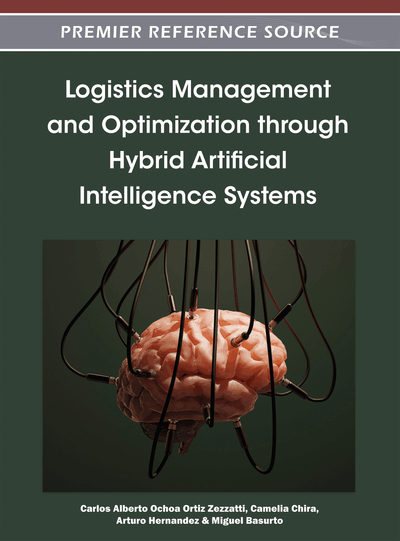# Looking for Reverse Transformations between NP-Complete Problems

Rodolfo A.Pazos R. (Rodolfo A. Pazos R.Instituto Tecnológico de Cd. Madero, Mexico), Ernesto Ong C. (Ernesto Ong C.Instituto Tecnológico de Cd. Madero, Mexico), Héctor Fraire H. (Héctor Fraire H.Instituto Tecnológico de Cd. Madero, Mexico), Laura Cruz R. (Laura Cruz R.Instituto Tecnológico de Cd. Madero, Mexico) and José A.Martínez F. (José A. Martínez F.Instituto Tecnológico de Cd. Madero, Mexico)
DOI: 10.4018/978-1-4666-0297-7.ch007

## Abstract

The theory of NP-completeness provides a method for telling whether a decision/optimization problem is “easy” (i.e., it belongs to the P class) or “difficult” (i.e., it belongs to the NP-complete class). Many problems related to logistics have been proven to belong to the NP-complete class such as Bin Packing, job scheduling, timetabling, etc. The theory predicts that for any pair of NP-complete problems A and B there must exist a polynomial time transformation from A to B and also a reverse transformation (from B to A). However, for many pairs of NP-complete problems no reverse transformation has been reported in the literature; thus the following question arises: do reverse transformations exist for any pair of NP-complete problems? This chapter presents results on an ongoing investigation for clarifying this issue.
Chapter Preview
Top

## 1. Introduction

Nowadays, logistics is an important issue within modern-day organizations so much so that departments are devoted exclusively to it. The logistic process involves packaging and another tasks to manage the flow of goods and services required to satisfy the requirements of customers. The Bin Packing problem (BPP) is a classical problem related with the space minimization of bins or boxes. BPP is widely used to develop applications in logistics, mainly in production and distribution tasks.

Some applications are naturally packing problems. Others are not, but are modeled artificially as such. Examples of the first class are: loading of bottle products into vehicles (Gonzalez-Barbosa et al., 2010), allocating a stack in a block for storage yards of container terminals (Murty, 2007). Among the applications of the second class are the following: selecting the providers of logistics services and the type and quantities required (Crainic et al., 2010), automobile sheet metal forming processes (Sathe et al., 2009), positioning of a set of circuit modules on a VLSI chip or on an FPGA for executing real-time software (Natale & Bini, 2007).

The theory of NP-completeness is a branch of the theory of computation that aims at determining how complex an algorithm has to be depending on the decision/ optimization problem to be solved by the algorithm; in simple words, the theory of NP-completeness provides a method for telling whether a problem is “easy” (i.e., it belongs to the P class) or “difficult” (i.e., it belongs to the NP-complete class).

Many problems related to logistics have been proven to belong to the NP-complete class such as the Bin Packing problem, the Knapsack problem, job scheduling, time-tabling, etc. Examples of problems that belong to the P class are Minimum Spanning Tree, Shortest Path, Minimum Cut, Sequencing with Deadlines, etc. (Karp, 1972). The procedure for classifying problems into the P or NP-complete classes is rather complex and it is briefly described in Section “Background.”

The classification of a problem as NP-complete (difficult) or P (easy) has a practical implication: for NP-complete problems no algorithm has been found (and there is little hope to find one) that can solve them in polynomial time. This means (in simple terms) that the time needed to obtain an exact solution for large instances of a problem (which arise in many practical applications) can take an extremely long time, way too much more than users are willing to wait for a solution. For large instances of NP-complete problems, it is advisable to use heuristic algorithms, which can produce fairly good solutions in a reasonable time.

The most widespread use of transformations between decision problems has been for proving that some given problem П1 is NP-complete (i.e., difficult) based on the known NP-completeness property of some other problem П2. However, transformations are also useful for: adapting an algorithm that solves problem П1 so it can be used for solving a similar problem П2, and extrapolating some knowledge on problem П1 to a similar problem П2.

More specifically, the practical usefulness of transformations between optimization problems, resides in the fact that if there exists an efficient solution algorithm for one of the problems, the instances of another problem can be solved using a transformation from this problem to the first one.

In Mahajan et al. (2005) the authors remark that “The emergence of efficient SAT solvers which can handle large structured SAT instances has enabled the use of SAT solvers in diverse domains such as verification, planning, routing, etc.” For example, many problems related to logistics, such as planning (Kautz & Selman, 1999; Xing et al., 2006), packing (Grandcolas & Pinto, 2010) and job scheduling (Ohrimenko et al., 2009) problems are transformed to the SATISFIABILITY problem for taking advantage of SAT solvers. In the domain of timetabling problems, a transformation to the graph coloring problem is often carried out followed by a transformation to SAT (Erben, 2001).

## Complete Chapter List

Search this Book:
Reset